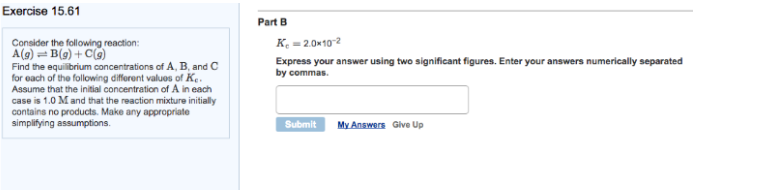# Problem: Consider the following reaction: A (g) ⇌ B (g) + C (g) Find the equilibrium concentrations of A, B, and C for each of the following different values of Kc. Assume that the initial concentration of A in each case is 1.0 M and that the reaction mixture initially contains no products. Make any appropriate simplifying assumptions. Part BKc = 2.0 x 10-2 Express your answer using two significant figures. Enter your answers numerically separated by commas.

###### FREE Expert Solution
95% (10 ratings)###### Problem Details

Consider the following reaction:

A (g) ⇌ B (g) + C (g)

Find the equilibrium concentrations of A, B, and C for each of the following different values of Kc. Assume that the initial concentration of A in each case is 1.0 M and that the reaction mixture initially contains no products. Make any appropriate simplifying assumptions.

Part B

Kc = 2.0 x 10-2